# BE Civil Engineering Semester 1 (FE First Year) - University of Mumbai Important Questions for Engineering Mechanics

Subjects
Topics
Subjects
Popular subjects
Topics
Engineering Mechanics
< prev  1 to 20 of 84  next >

state and prove varigon’s theorem.

Appears in 1 question paper
Chapter:  System of Coplanar Forces
Concept: Varignon’s Theorem

Find the resultant of the force system as shown in the given figure.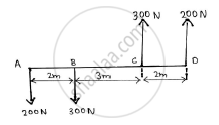Appears in 1 question paper
Chapter:  System of Coplanar Forces
Concept: Resultant of concurrent forces

Find the resultant of the force acting on the bell crank lever shown. Also locate its position with respect to hinge B.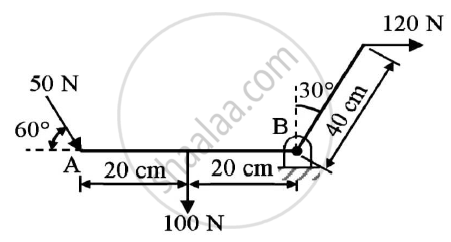Given : Forces on the bell crank lever
To find : Resultant and it’s position w.r.t hinge B

Appears in 1 question paper
Chapter:  System of Coplanar Forces
Concept: Resultant of parallel forces

In the rocket arm shown in the figure the moment of ‘F’ about ‘O’ balances that P=250 N.Find F.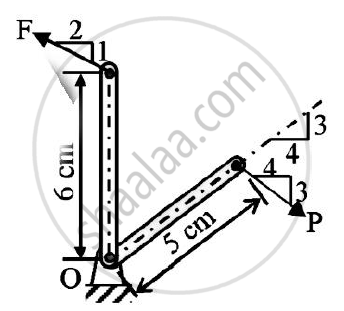Appears in 1 question paper
Chapter:  System of Coplanar Forces
Concept: Resultant of concurrent forces

Find the force F4 , so as to give the resultant of the force as shown in the figure given below.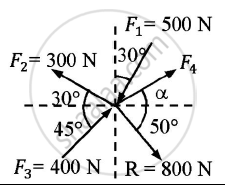Given : Forces and their resultant
To find : Force F4

Appears in 1 question paper
Chapter:  System of Coplanar Forces
Concept: Resultant of concurrent forces

The resultant of the three concurrent space forces at A is 𝑹̅ = (-788𝒋̅) N. Find the magnitude of F1,F2 and F3 forces.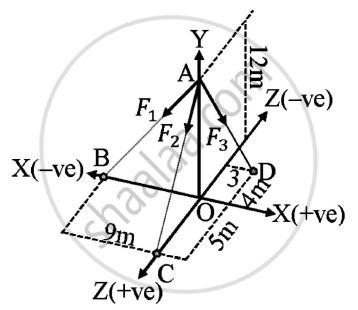Given : A=(0,12,0)
B=(-9,0,0)
C=(0,0,5)
D=(3,0,-4)
Resultant of forces = (-788𝑗̅) N
To find : Magnitude of forces F1,F2,F3

Appears in 1 question paper
Chapter:  System of Coplanar Forces
Concept: Resultant of concurrent forces

A force of 140 kN passes through point C (-6,2,2) and goes to point B (6,6,8). Calculate moment of force about origin.

Appears in 1 question paper
Chapter:  System of Coplanar Forces
Concept: Moment of force about a point

A machine part is subjected to forces as shown.Find the resultant of forces in magnitude and in direction.
Also locate the point where resultant cuts the centre line of bar AB.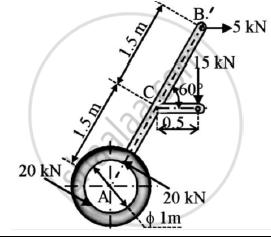Solution:
Given : A machine subjected to various forces
To find : Resultant of forces
Point where the resultant force cuts the bar AB

Appears in 1 question paper
Chapter:  System of Coplanar Forces
Concept: Varignon’s Theorem

Find the resultant of the parallel force system shown in Figure 1 and locate the same with respect to point C.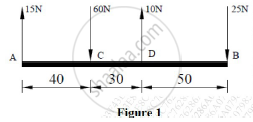Appears in 1 question paper
Chapter:  System of Coplanar Forces
Concept: Resultant of parallel forces

A force of magnitude of 20kN, acts at point A(3,4,5)m and has its line of action passing through B(5,-3,4)m. Calculate the moment of this force about a line passing through points S(2,-5,3) m and T(-3,4,6)m.

Appears in 1 question paper
Chapter:  System of Coplanar Forces
Concept: Moment of force about a point

Determine the position of the centroid of the plane lamina. Shaded portion is removed.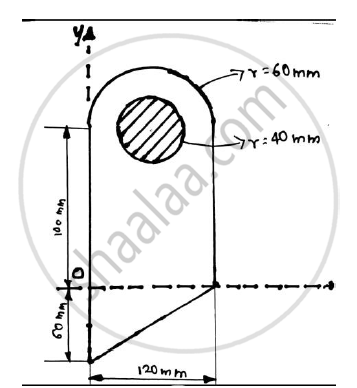Appears in 1 question paper
Chapter:  Center of Gravity and Centroid for Plane Laminas
Concept: Centroid for Plane Laminas

Find the centroid of the shaded portion of the plate shown in the figure.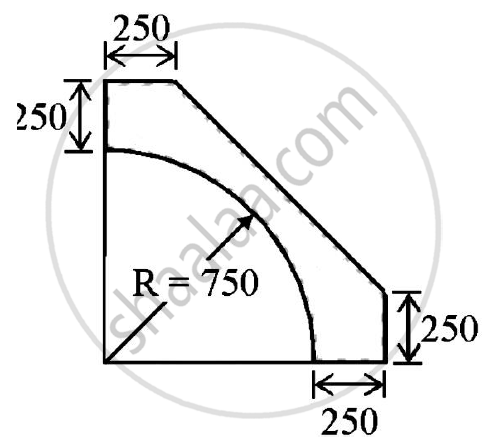Appears in 1 question paper
Chapter:  Center of Gravity and Centroid for Plane Laminas
Concept: Centroid for Plane Laminas

For the composite lamina shown in the figure, determine the coordinates of its centroid.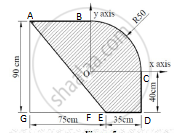Appears in 1 question paper
Chapter:  Center of Gravity and Centroid for Plane Laminas
Concept: Centroid for Plane Laminas

Determine the reaction at points of constant 1,2 and 3. Assume smooth surfaces.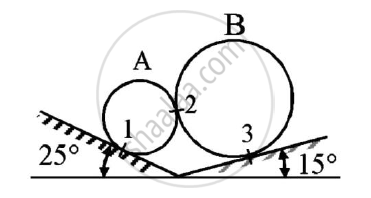Given: The spheres are in equilibrium
To find: Reactions at points 1,2 and 3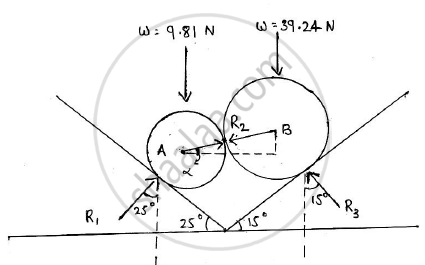Appears in 1 question paper
Chapter:  Equilibrium of System of Coplanar Forces
Concept: Condition of Equilibrium for non-concurrent nonparallel general forces

Explain the conditions for equilibrium of forces in space.

Appears in 1 question paper
Chapter:  Equilibrium of System of Coplanar Forces
Concept: Condition of equilibrium for concurrent forces

Find the support reactions at A and B for the beam loaded as shown in the given figure.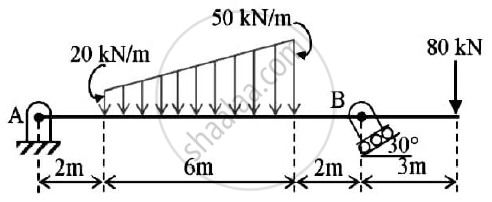Appears in 1 question paper
Chapter:  Equilibrium of System of Coplanar Forces
Concept: Condition of equilibrium for parallel forces

Determine the force P required to move the block A of 5000 N weight up the inclined plane, coefficient of friction between all contact surfaces is 0.25. Neglect the weight of the wedge and the wedge angle is 15 degrees.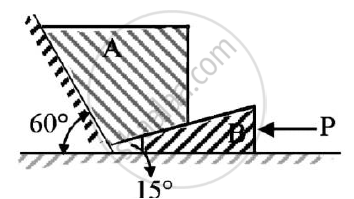Given : Weight of block A = 5000 N
μs=0.25
Wedge angle = 15º

To find : Force P required to move block A up the inclined plane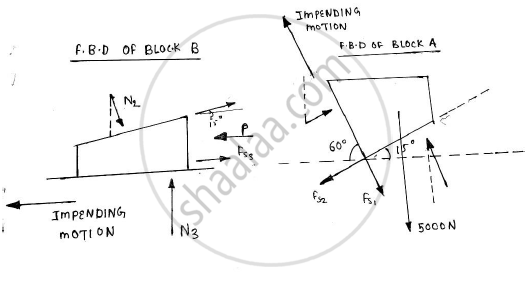Appears in 1 question paper
Chapter:  Equilibrium of System of Coplanar Forces
Concept: Condition of equilibrium for concurrent forces

State Lami’s theorem.
State the necessary condition for application of Lami’s theorem.

Appears in 1 question paper
Chapter:  Equilibrium of System of Coplanar Forces
Concept: Condition of equilibrium for parallel forces

Two spheres A and B of weight 1000N and 750N respectively are kept as shown in the figure..Determine reaction at all contact points 1,2,3 and 4. Radius of A is 400 mm and radius of B is 300 mm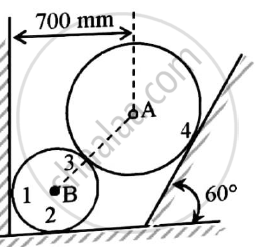Given  : Two spheres are in equilibrium
W1=1000 N
W2=750 N
rA=400 mm
rB=300 mm
To find : Reaction forces at contact points 1,2,3 and 4

Appears in 1 question paper
Chapter:  Equilibrium of System of Coplanar Forces
Concept: Condition of Equilibrium for non-concurrent nonparallel general forces

Refer to figure.If the co-efficient of friction is 0.60 for all contact surfaces and θ = 30o,what force P applied to the block B acting down and parallel to the incline will start motion and what will be the tension in the cord parallel to inclined plane attached to A.
Take WA=120 N and WB=200 N.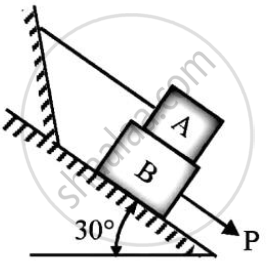Appears in 1 question paper
Chapter:  Equilibrium of System of Coplanar Forces
Concept: Condition of equilibrium for concurrent forces
< prev  1 to 20 of 84  next >